Next: About this document ... Up: lab_template Previous: lab_template

Subsections

# Double integrals with Maple

## Purpose

The purpose of this lab is to acquaint you with using Maple to do double integrals.

## Getting Started

To assist you, there is a worksheet associated with this lab that contains examples and even solutions to some of the exercises. You can copy that worksheet to your home directory by going to your computer's Start menu and choose run. In the run field type:

\\filer\calclab


when you hit enter, you can then choose MA1024 and then choose the worksheet

Doubleint_start_B11.mw


Remember to immediately save it in your own home directory. Once you've copied and saved the worksheet, read through the background on the internet and the background of the worksheet before starting the exercises.

## Background

### Volumes from double integrals

Suppose that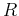is a rectangular region in the the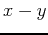plane, and that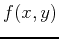is a continuous, non-negative function on. Then the volume of the solid aboveand below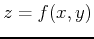is given by the double integral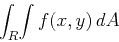You learned in class that such integrals can be evaluated by either of the iterated integrals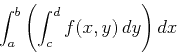orwhere the rectangleis defined by the inequalitiesand.

The worksheet associated with this lab contains examples of how to use Maple to compute double integrals. It also has an example of how to use Maple if the region of integration is of the more complicated form. This is the case where the base of the solid is not rectangular, but is bounded by two curves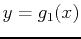and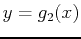. Ifis as before, then the volume of the solid aboveand belowis given by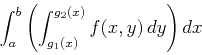The other case, where the regionis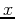-simple can also be handled using Maple, and there is an example in the worksheet.

## Exercises

1. Use Maple to compute the following double integrals.
a)b)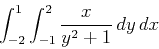c)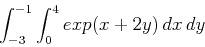2. Letbe the region in theplane bounded by the two curves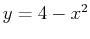and. Use a double integral to compute the area of the region. Use both orders of integration. Include a plot of the regionin your worksheet.

3. Use a double integral to find the volume of the region bounded by the two paraboloids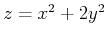and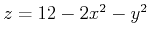.

4. Use a double integral to prove that the volume of a sphere of radius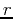is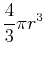.Next: About this document ... Up: lab_template Previous: lab_template
Dina J. Solitro-Rassias
2011-10-24